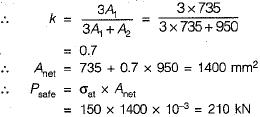Courses

# Tension Members - 1

## 10 Questions MCQ Test Mock Test Series for Civil Engineering (CE) GATE 2020 | Tension Members - 1

Description
This mock test of Tension Members - 1 for Civil Engineering (CE) helps you for every Civil Engineering (CE) entrance exam. This contains 10 Multiple Choice Questions for Civil Engineering (CE) Tension Members - 1 (mcq) to study with solutions a complete question bank. The solved questions answers in this Tension Members - 1 quiz give you a good mix of easy questions and tough questions. Civil Engineering (CE) students definitely take this Tension Members - 1 exercise for a better result in the exam. You can find other Tension Members - 1 extra questions, long questions & short questions for Civil Engineering (CE) on EduRev as well by searching above.
QUESTION: 1

### Consider the following statements regarding tensile test diagrams for carbon steels with varying carbon contents: As the carbon content increases 1. the ultimate strength of steel decreases 2. the elongation before fracture increases 3. the ductility of the metal decreases 4. the ultimate strength increases Which of these statements are correct?

Solution:

Carbon has the maximum influence on the mechanical properties of steel. Iron-carbon alloys containing up to 2 per cent carbon are called carbon steel while those having more than 2% are called cast steel. With increase in carbon the tensile strength of steel increases but the ductility falls.

QUESTION: 2

### The effective flange area in tension of a piate girder is equal to where Af is the area of each flange and Aw is the web area.

Solution:

Effective flange area =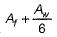Net area of the flange =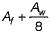QUESTION: 3

### The order of elongation which a specimen of mild steel undergoes before fracture is

Solution:

For mild steel
(i) Proportional limit (190 - 220) N/mm2
(ii) Yield strength (230 - 250) N/mm2
(iii) Ultimate strength (410 - 530) N/mm2
(iv) Fracture strength (250 - 300) N/mm2
(v) Elongation at fracture (23 - 35)% Thus the order of elongation is 10%

QUESTION: 4

A plate used for connecting two or more structural members intersecting each other is termed as

Solution:

Base plates are provided to distribute the load of a column on greater area.

QUESTION: 5

Which one of the following stresses is independent of yield stress as a permissible stress for steel members?

Solution:

Permissible values
Axial tensile stress = 0.6fy
Bearing stress = 0.75fy
Maximum shear stress = 0.45fy
Stress in slab base = 185 MPa for all type of steels.

QUESTION: 6

An equal angle of area A has been attached to the support by means of a lug angle. If allowable stress in tension is f, what is the load carrying capacity of the member?

Solution:

Strength of member = allowable tensile stress x
Net area of the angle
Net area = Gross Area - deduction for holes.

QUESTION: 7

The effective length of an angle member in a riveted truss is equal to which one of the following?

where I is the centre to centre distance between the joints.

Solution: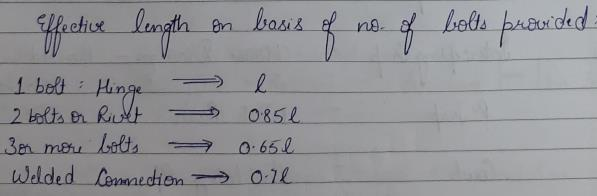QUESTION: 8

Steel of yield strength 400 MPa has been used in a structure. What is the value of the maximum allowable tensile strength?

Solution:

Maximum allowable tensile strength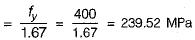QUESTION: 9

A steel plate is 300 mm wide and 10 mm thick. A rivet of nominal diameter 18 mm is driven. The net sectional area of the plate is

Solution:

Net sectional area of the plate is given by,
Anet = (B - nd) x t
= [300-1 x (18 + 1.5)] x 10
= 2805 mm2

QUESTION: 10

The capacity of a single ISA 100 x 100 x 10 mm as tension member connected by one leg only using 6 rivets of 20 mm diameter is

The allowable stress is 150 N/mm2

Solution:

The net area of the tension member is given by,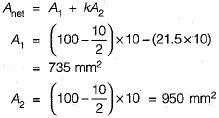The angle section is connected by one leg only.